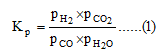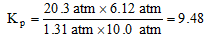# The chemical equation for the water-gas shift reaction is CO (g) + H2O (g) ⇌ CO2 (g) + H2 (g). What is the value of molal equilibrium constant Kp at 700 K if the partial pressures in an equilibrium mixture at 700 K are 1.31 atm of CO, 10.0 atm of water, 6.12 atm of carbon dioxide, and 20.3 atm of hydrogen gas?

Question-AnswerCategory: Chemical ThermodynamicsThe chemical equation for the water-gas shift reaction is CO (g) + H2O (g) ⇌ CO2 (g) + H2 (g). What is the value of molal equilibrium constant Kp at 700 K if the partial pressures in an equilibrium mixture at 700 K are 1.31 atm of CO, 10.0 atm of water, 6.12 atm of carbon dioxide, and 20.3 atm of hydrogen gas?

The chemical equation for the water-gas shift reaction is CO (g) + H2O (g) ⇌ CO2 (g) + H2 (g). What is the value of molal equilibrium constant Kp at 700 K if the partial pressures in an equilibrium mixture at 700 K are 1.31 atm of CO, 10.0 atm of water, 6.12 atm of carbon dioxide, and 20.3 atm of hydrogen gas?

Given:
Partial pressure of CO = 1.31 atm
Partial pressure of water = 10.0 atm
Partial pressure of carbon dioxide = 6.12 atm
Partial pressure of hydrogen gas = 20.3 atm

Step 2

The ratio of mathematical product of equilibrium partial pressure of products to the mathematical product of equilibrium partial pressure of reactants raised to power equal to their stoichiometric coefficient in the balanced chemical equation is known as equilibrium constant (Kp).
The given reaction is:
CO(g) + H2O(g) ⇌ CO2(g) + H2(g)
The equilibrium constant (Kp) expression for the reaction is:Substitute the given values in equation (1).Therefore, the value of molal equilibrium constant Kp at 700 K is 9.48.